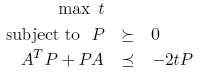# Decay rate computation in LTI system

Tags:

Updated:

This example is motivated by one of the most asked question: How can I do GEVP optimization?

The short answer is that YALMIP does not have a ready-to-run script for solving generalized eigenvalue problems, such as the gevp command in LMILAB.

However, luckily this is not entirely true, and the long answer is given by this example.

The problem we will solve is to estimate the decay-rate of a linear system $$\dot{x}= Ax$$. This can be formulated as a generalized eigenvalue problem.Due to the product between $$t$$ and $$P$$, the problem cannot be solved directly using linear semidefinite programming. Effectively it is a BMI (bilinear matrix inequality) which generically are intractable, but luckily it is not that bad here.

### Bisection algorithm

The problem is not linear, but it is easily seen to be quasi-convex (the feasible set scales monotonically in $$t$$). Hence we can solve it by bisection in $$t$$. We will first implement a bisection manually, and then use the built-in YALMIP meta-solver bisection.

Simple description of a bisection approach

1. Find a lower bound on optimal $$t$$ (any feasible $$t$$ you can compute)
2. Find an upper bound on optimal $$t$$ (e.g., increase the lower bound until a feasibility problem for fixed $$t$$ is infeasible).
3. Start bisection, i.e., check value between lower and upper bound. If feasible, update lower bound, if infeasible update upper bound. Repeat until bounds are sufficiently close.

Define the variables.

A = [-1 2;-3 -4];
P = sdpvar(2,2);


To find a lower bound on $$t$$, we solve a standard Lyapunov stability problem.

F = [P>=eye(2), A'*P+P*A <= -eye(2)];
optimize(F,trace(P));
P0 = value(P);


In the code above, we minimized the trace just to get a numerically sound solution. This solution gives us a lower bound the attainable decay rate, i.e. a feasible but not necessarily optimal value.

t_lower = -max(eig(inv(P0)*(A'*P0+P0*A)))/2;


Alternatively, we could just as well have picked the lower bound as 0, it would only mean that we would have to run the bisection algorithm for a couple more iterations.

We now find an upper bound on the decay-rate by doubling $$t$$ until the problem is infeasible. To find out if the problem is infeasible, we check the field problem in the solution structure. The meaning of this variable is explained in the help text for the command yalmiperror. Infeasibility has been detected by the solver if the value is 1. To reduce the amount of information written on the screen, we run the solver in a completely silent mode. This can be accomplished by using the verbose and warning options in sdpsettings.

t_upper = t_lower*2;
F = [P>=eye(2), A'*P+P*A <= -2*t_upper*P];
ops = sdpsettings('verbose',0,'warning',0);
sol = optimize(F,[],ops);
while ~(sol.problem==1)
t_upper = t_upper*2;
F = [P>=eye(2), A'*P+P*A <= -2*t_upper*P];
sol = optimize(F,[],ops);
end


Having both an upper bound and a lower bound allows us to perform a bisection.

tol = 0.01;
t_works = t_lower
while (t_upper-t_lower)>tol
t_test = (t_upper+t_lower)/2;
disp([t_lower t_upper t_test])
F = [P>=eye(2), A'*P+P*A <= -2*t_test*P];
sol = optimize(F,[],ops);
if sol.problem==1
t_upper = t_test;
else
t_lower = t_test;
t_works = t_test;
Pworks = value(P);
end
end


Of course, in a real case, the code should be extended with more analysis of the returned error code (to check for numerical problems etc).

### Faster code through optimizer

By using the optimizer construct, we can get rid of most of the YALMIP overhead. We create an optimizer object which solves the feasibility SDP for particular values of $$t$$ (since the optimizer requires an explicit solver choice for nonlinearly parameterized models, the code below is hard-coded for MOSEK)

t_lower = 0;
t_upper = 1;
sdpvar t
ops = sdpsettings('solver','mosek');
Constraints = [P>=eye(2), A'*P+P*A <= -2*t*P];
Solver = optimizer(Constraints,[],ops,t,P);
[~, errorcode] = Solver{t_upper};
while ~(errorcode==1)
t_upper = t_upper*2;
[~, errorcode] = Solver{t_upper};
end

t_works = t_lower;
while (t_upper-t_lower)>tol
t_test = (t_upper+t_lower)/2;
disp([t_lower t_upper t_test])
[Popt, errorcode] = Solver{t_test};
if errorcode==1
t_upper = t_test;
else
t_lower = t_test;
t_works = t_test;
Pworks = Popt;
end
end


### Built-in bisection solver

The bisection algorithm above has been implemented in a high-level solver bisection,

sdpvar t
Constraints = [P>=eye(2), A'*P+P*A <= -2*t*P];
Objective = -t;
diagnostics = bisection(Constraints, Objective,sdpsettings('solver','mosek'))


Alternatively, call it as any other solver

opssdpsettings('solver','bisection','bisection.solver','mosek');
diagnostics = optimize(Constraints, Objective,ops)


### Nonlinear SDP solver

An alternative way to solve the problem is to install the nonlinear semidefinite programming solvers PENBMI or PENLAB. These solvers will easily solve the problem, and is guaranteed (in theory…) to find the global optima due to the quasi-convexity.

t = sdpvar(1);
F = [P >= eye(2), A'*P+P*A <= -2*t*P];
optimize(F,-t,sdpsettings('solver','penlab'));


### Global SDP solver

If PENBMI or PENLAB is installed, the decay-rate problem should easily be solved, in theory. In practice, the solver may encounter numerical problems. An alternative then is to run YALMIPs internal global SDP solver BMIBNB. This is a bit of an over-kill, but it is convenient, compared to writing the script above, and is actually just as fast.

F = [P>=0, A'*P+P*A <= -2*t*P, trace(P)== 1, 100 >= t >= 0];
optimize(F,-t,sdpsettings('solver','bmibnb'));


In this model, we have changed the constraints slightly, in order to ensure a bounded search-space. For details, check out the [global optimization tutorial].

The code here still assumes you have a nonlinear semidefinite solver installed, since a local solver is needed during the branch and bound search. If you do not have one installed, you can tell YALMIP to perform the branch and bound without any local solver (upper bounds will then be generated using trivial heuristics from relaxed solutions)

optimize(F,-t,sdpsettings('solver','bmibnb','bmibnb.upper','none'));


This will not perform as well though, and a better choice is to use the bisection approach.